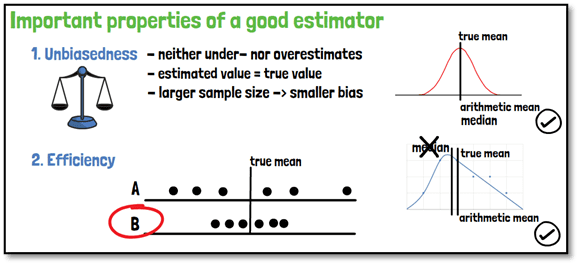# What is an estimator?An example for a point estimator is the arithmetic mean when trying to find the mean of a population. A point estimator is used to infer the value of an unknown parameter in a statistical model, and it yields a single value.

The rule or function that is used to create the estimate is the estimator. Therefore, the result is the estimate. It is based on a given quantity based on observed data. The quantity of interest or the parameter investigated is called estimand. An estimand has several characteristics, that are describing exactly the aim of the estimation.

Interval estimators give you not only a single value, but a range of plausible values. One example is the confidence interval. The results of an interval estimator are referred to as interval estimates.

Two very important properties of a good estimator are unbiasedness and efficiency.

1. Unbiasedness: An accurate estimate is one that neither under- nor overestimates. That is given, if the estimated population parameter is identical to the true value. With a large sample size, the bias of the estimator is reduced to a minimum. For example, the arithmetic mean is an unbiased estimator for the true mean, independent of the distribution of the sample, whereas the median is an unbiased estimator of the expected mean only if the population distribution is symmetrical. If the distribution is skewed, the sample mean would be the better choice in terms of unbiasedness.
2. Efficiency: If two estimators are unbiased, the estimate with the smaller variance for the given sample size is more efficient. Because if the variance is small, it means that the distribution is more concentrated around the parameter estimated, and therefore the quality of that estimator is better. An example would be the mean in a symmetrical distribution.

If you are interested in a visual presentation of this topic, please feel free to follow us on our GCP Mindset YouTube channel! If you would like to know more about how we could implement statistics in your clinical trial, send us a mail to statistics@gcp-service.com.